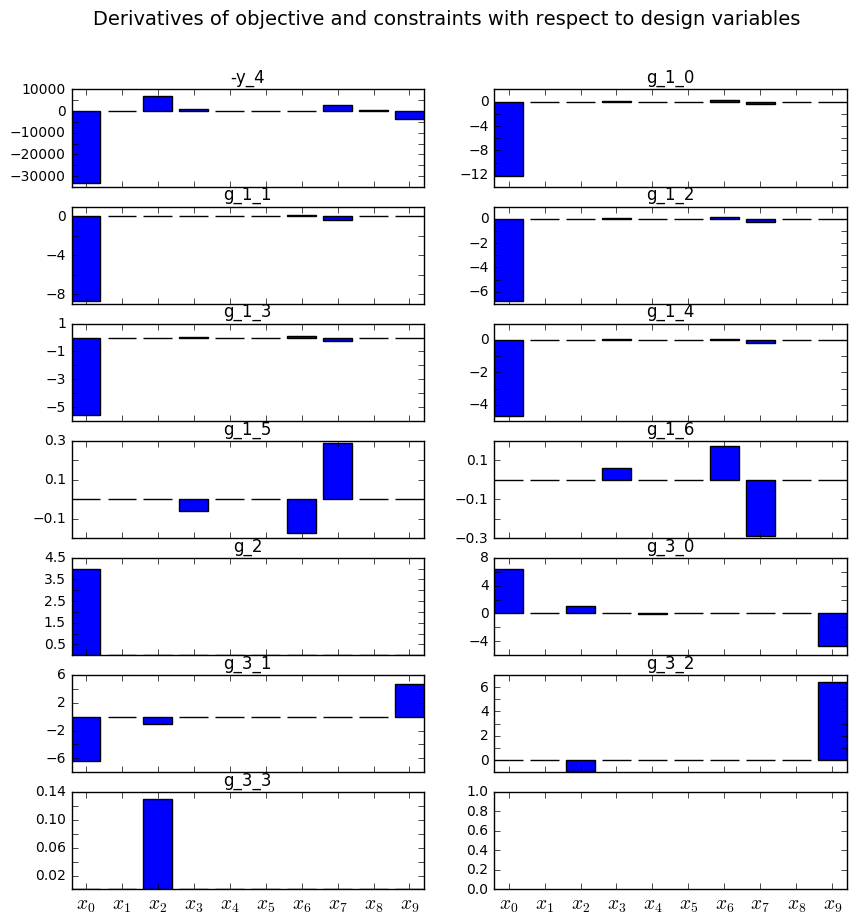## Preliminaries: instantiation and execution of the MDO scenario¶

Let’s start with the following code lines which instantiate and execute the MDOScenario :

from gemseo.api import create_discipline, create_scenario

formulation = 'MDF'

disciplines = create_discipline(["SobieskiPropulsion", "SobieskiAerodynamics",
"SobieskiMission", "SobieskiStructure"])

scenario = create_scenario(disciplines,
formulation=formulation,
objective_name="y_4",
maximize_objective=True,
design_space="design_space.txt")

scenario.set_differentiation_method("user")

algo_options = {'max_iter': 10, 'algo': "SLSQP"}
for constraint in ["g_1","g_2","g_3"]:

scenario.execute(algo_options)


### Description¶

The GradientSensitivity post processing builds histograms of derivatives of objective and constraints

The plot method considers the derivatives at the last iteration. The iteration can be changed in option. The x- and y- figure sizes can also be modified in option. It is possible either to save the plot, to show the plot or both.

### Options¶

• extension, str - file extension

• figsize_x, int - size of figure in horizontal direction (inches)

• figsize_y, int - size of figure in vertical direction (inches)

• file_path, str - the base paths of the files to export

• iteration, int - the iteration to plot sensitivities, if negative, use optimum

• save, bool - if True, exports plot to pdf

• show, bool - if True, displays the plot windows

## Case of the MDF formulation¶

To visualize the gradient sensitivity plot of the scenario, we use the execute_post() API method with the keyword "GradientSensitivity" and additional arguments concerning the type of display (file, screen, both):

scenario.post_process(“GradientSensitivity”, save=True, show=False,file_path=“mdf” )


The figure Gradient sensitivity on the Sobieski use case for the MDF formulation shows the total derivatives of the objective and constraints with respect to the design variables: $$\frac{d f}{d x_i}$$:

• a large value means that the function is sensitive to the variable,

• a null value means that, at the optimal solution, the function does not depend on the variable,

• a negative value means that the function decreases when the variable is increased.Gradient sensitivity on the Sobieski use case for the MDF formulation

$$x_0$$ (wing-taper ratio) and $$x_2$$ (Mach number) appear to be the most important for the gradient of the objective function.

The g_1_0 to g_1_4 are very similar, since they all quantify the stress in various sections. g_1_5 and g_1_6 correspond to the lower and upper bounds of the twist , therefore their sensitivities are opposite. g_2 is a function of only $$x_0$$ ; $$x_0$$ is the only variable that influences its gradient.